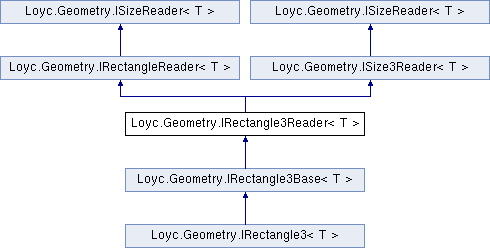Enhanced C# Language of your choice: library documentation

# Documentation moved to ecsharp.net

GitHub doesn't support HTTP redirects, so you'll be redirected in 3 seconds.

Loyc.Geometry.IRectangle3Reader< T > Interface Template Reference

Represents a read-only 3D rectangular prism. More...

Source file:
Inheritance diagram for Loyc.Geometry.IRectangle3Reader< T >:## Remarks

Represents a read-only 3D rectangular prism.

The relationship between Z1, Z2 and Depth, as well as between the other coodinates and Width/Height, depends on whether the object represents a starting point plus a size, or a bounding rectangle or a pair of points. Either the object stores a starting point (X1, Y1, Z1) and a size (Width, Height, Depth), or it stores a starting point and an ending point (X2, Y2, Z2).

Conventionally, when using the size representation, the Width is defined as X2 - X1, the Height as Y2 - Y1, and the Depth as Z2 - Z1. A bounding rectangle is defined slightly differently: the Width is X2 - X1 + e, the Height is Y2 - Y1 + e, and the Depth is Z2 - Z1 + e, where e is an infitessimal value of type T (e=1 if T is an integer type).

Finally, this interface could simply represent a pair of points. In that case, Width and Height return the absolute value of X2-X1 and Y2-Y1 respectively.

The object may or may not require X2 >= X1 and Y2 >= Y1 and Z2 >= Z1. If X1>X2 or Y1>Y2 or Z1>Z2, the rectangle is said to be "not normalized" and the Rectangle3Ext.IsNormal extension method returns false.

## Properties

Z1` [get]`

Z2` [get]`Properties inherited from Loyc.Geometry.IRectangleReader< T >
X1` [get]`

Y1` [get]`

X2` [get]`

Y2` [get]`Properties inherited from Loyc.Geometry.ISizeReader< T >
Width` [get]`
Gets the width of a rectangle (the difference between X coordinates). More...

Height` [get]`
Gets the height of a rectangle (the difference between Y coordinates). More...Properties inherited from Loyc.Geometry.ISize3Reader< T >
Depth` [get]`
Gets the depth of a rectangle (the difference between Z coordinates). More...